# CirclesPage 1

#### WATCH ALL SLIDES

Slide 1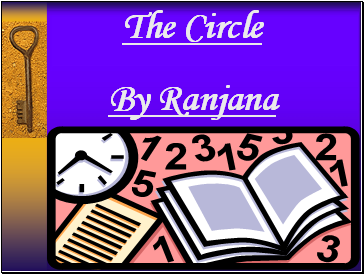The Circle

By Ranjana

Slide 2## Circle

O

A

Eg. ball,bangle,lemon.coin

Slide 3O

A

CENTRE : O

B

CENTRE

Slide 4.

O

In a plane,each point of the circle is at equal distance from a fixed point.The fixed point is called the centre of the circle.

CENTRE = O

Slide 5O

The distance from centre to any point on the circle is called radius of the circle.

A

Slide 6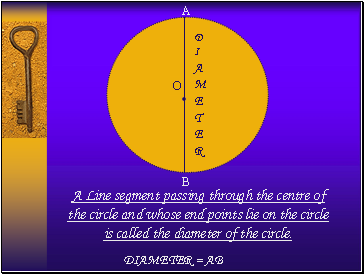.

O

DI AMETER

A Line segment passing through the centre of the circle and whose end points lie on the circle is called the diameter of the circle.

A

B

DIAMETER = AB

Slide 7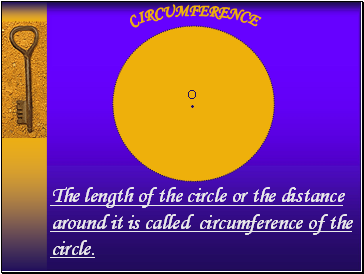## Circumference

.

O

The length of the circle or the distance around it is called circumference of the circle.

Slide 8.

O

A

B

A Line segment whose both the ends touches the circumference and passes through the centre is called diameter.

DIAMETER : AB

Slide 9## Relation between radius and diameter

.

O

A

B

2

OB = AB

2

Slide 10Eg. If diameter of the circle is 10 cm then find its radius?

.

O

Diameter = 10cm

A

B

Slide 11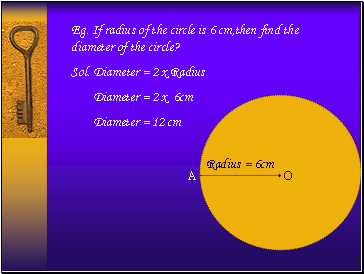.

O

Eg. If radius of the circle is 6 cm,then find the diameter of the circle?

A

Sol. Diameter = 2 x Radius

Diameter = 2 x 6cm

Diameter = 12 cm

Slide 12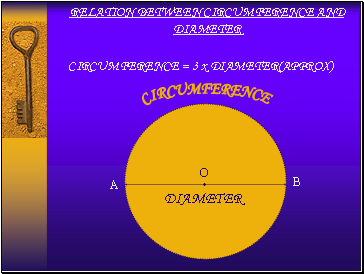## Relation between circumference and diameter

.

O

CIRCUMFERENCE

DIAMETER

CIRCUMFERENCE = 3 x DIAMETER(APPROX)

A

B

Slide 13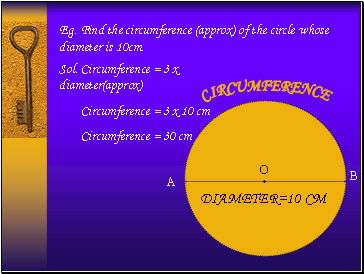Eg. Find the circumference (approx) of the circle whose diameter is 10cm.

.

O

CIRCUMFERENCE

DIAMETER=10 CM

Sol. Circumference = 3 x diameter(approx)

Go to page:
1  2